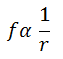Please visit, subscribe and share 10 Minutes Lectures in Computer Science

## Natural Language Processing MCQ with answers

1. Given a sequence of observations and a HMM model, which of the following fundamental problems of HMM finds the most likely sequence of states that produced the observations in an efficient way?
(a) Evaluation problem
(b) Likelihood estimation problem
(c) Decoding problem
(d) Learning problem

### 2. Zipf’s law states that

(a) The frequency of a word type is proportional to its rank by frequency.
(b) The frequency of a word type is inversely proportional to its rank by frequency.
(c) The frequency of a word type does not influence its ranking.
(d) None of the above
 Answer: (b) Zipf’s law – If f is the frequency of word type in a large corpus, and r is the rank (All word types in the corpus are arranged in the order of frequency of occurrence, from most to less frequent. The position of word r in this list is the rank) then,It is the commonly used model of the distribution of terms in a collection.

3. Which of the following is not a problem when using Maximum Likelihood Estimation to obtain the parameters in a language model?
(a) Out-of-vocabulary items
(b) Over-fitting
(c) Smoothing
(d) Unreliable estimates when there is little training data

### Smoothing is a normalization technique which is needed if MLE value calculated as 0.

4. The words “window” and “room” are in a lexical semantic relation
(a) hypernym – hyponym
(b) hypernym – meronym
(c) holonym – hyponym
(d) meronym – holonym
 Answer: (d) Meronym is a part of something and the holonym is the whole of something. In this question, window is the meronym of room (part of room) and room is the holonym of window (room consists of window).

5. In an HMM, observation likelihoods measure
(a) The likelihood of a POS tag given a word
(b) The likelihood of a POS tag given the preceding tag
(c) The likelihood of a word given a POS tag
(d) The likelihood of a POS tag given two preceding tags

***********